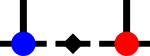SyTentemplate<Rank rank>
 bool syten::checkedAddParallel ( TensorBlock< rank > & a, TensorBlock< rank > & b, EliminateZeros const ezeros = EliminateZeros::Yes, bool add_cgc = false, bool no_zero_check_dense = false )
inline

Checks if a and b can be combined additively into a single block.

This is possible if either all CGC spaces are parallel or all but one CGC spaces are parallel and the reduced tensors are parallel.

In the former case, the reduced tensors will be added element-wise (after scaling to make the CGC spaces identical), in the latter case the non-parallel CGC space will be added elementwise (after scaling). That is, if we have:

$m_a \otimes c_{a_1} \otimes c_{a_2} + m_b \otimes c_{b_1} \otimes c_{b_2}$

and $$c_{b_1} = f_{c_1} c_{a_1}$$ and $$c_{b_2} = f_{c_2} c_{a_2}$$ , with $$f_x$$ scalar and real, we can instead write:

$\left[m_a + \left(m_b f_{c_1} f_{c_2}\right)\right] \otimes c_{a_1} \otimes c_{a_2} .$

Similarly, if say $$m_b = f_m m_a$$ and $$c_{b_2} = f_{c_2} c_{a_2}$$ , we have

$m_a \otimes \left[c_{a_1} + \left(c_{b_1} f_m f_{c_2}\right)\right] \otimes c_{a_2} .$

If more than one of the tensors are non-parallel, returns false. If the above rewriting can be done, it is done with the result in a, b set to zero and a return value of true.

Remarks
In principle, $$f_x$$ complex would not hurt. However, our CGC spaces are purely real at the moment, meaning that if we had a complex factor from the reduced tensors and then moved it into the CGC space, that CGC space would become complex as well. Bad.
We currently do not consider reduced tensors to be parallel, as this would introduce Scalars into the CGC spaces for rescaling, getting the nice multiprecision things in there all dirty. This can be overwritten by setting add_cgc to true, but it is likely really not advisable.
The EliminateZeros option is passed through to add_scaled.

Referenced by syten::Tensor< 6 >::add(), and syten::Tensor< 6 >::reduce().Here is the call graph for this function:Here is the caller graph for this function: